# Electronic Devices - Online Test

Q1. A p n junction has a built-in potential of 0.8 V. The depletion layer width a reverse bias of 1.2 V is 2 μm. For a reverse bias of 7.2 V, the depletion layer width will be
Explaination / Solution: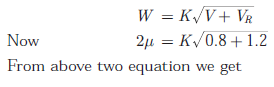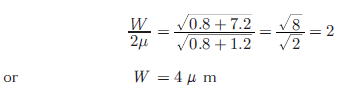Q2. Group I lists four types of p − n junction diodes. Match each device in Group I with one of the option in Group II to indicate the bias condition of the device in its normal mode of operation. Group - I Group-II (P) Zener Diode (1) Forward bias (Q) Solar cell (2) Reverse bias (R) LASER diode (S) Avalanche Photodiode
Explaination / Solution:

Zener diode and Avalanche diode works in the reverse bias and laser diode works in forward bias. In solar cell diode works in forward bias but photo current is in reverse direction. Thus Zener diode : Reverse Bias Solar Cell : Forward Bias Laser Diode : Forward Bias Avalanche Photo diode : Reverse Bias

Q3. The DC current gain (β) of a BJT is 50. Assuming that the emitter injection efficiency is 0.995, the base transport factor is
Explaination / Solution:Q4.
Group I lists four different semiconductor devices. match each device in Group I with its charactecteristic property in Group II
Group-I                                      Group-II
(P) BJT                                       (1) Population iniversion
(Q) MOS capacitor                     (2) Pinch-off voltage
(R) LASER diode                        (3) Early effect
(S) JFET                                     (4) Flat-band voltage
Explaination / Solution:

In BJT as the B-C reverse bias voltage increases, the B-C space charge region width increases which xB (i.e. neutral base width) > A change in neutral base width will change the collector current. A reduction in base width will causes the gradient in minority carrier concentration to increase, which in turn causes an increased in the diffusion current. This effect si known as base modulation as early effect.
In JFET the gate to source voltage that must be applied to achieve pinch off voltage is described as pinch off voltage and is also called as turn voltage or threshold voltage.
In LASER population inversion occurs on the condition when concentration of electrons in one energy state is greater than that in lower energy state, i.e. a non equilibrium condition.
In MOS capacitor, flat band voltage is the gate voltage that must be applied to create flat ban condition in which there is no space charge region in semiconductor under oxide.
Therefore
BJT : Early effect
MOS capacitor : Flat-band voltage
LASER diode : Population inversion
JFET : Pinch-off voltage

Q5. For the BJT circuit shown, assume that the β of the transistor is very large and VBE = . V. The mode of operation of the BJT isExplaination / Solution: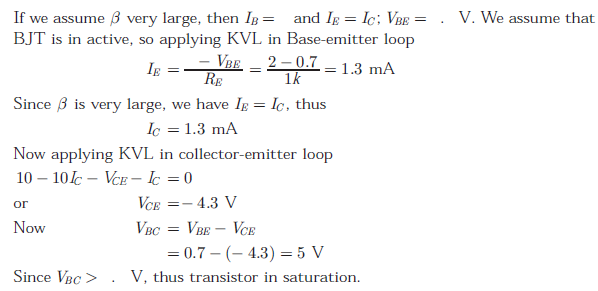Q6. In the CMOS inverter circuit shown, if the trans conductance parameters of the NMOS and PMOS transistors are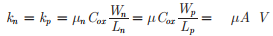and their threshold voltages ae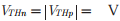the current I is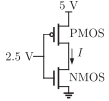Explaination / Solution: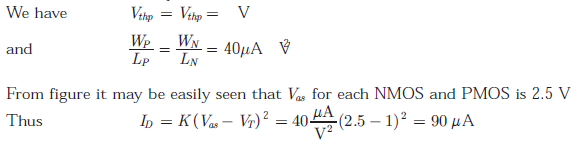Q7. The figure shows the high-frequency capacitance - voltage characteristics of Metal/Sio2/silicon (MOS) capacitor having an area of 1 × 10-4 cm2. Assume that the permittivities (ε0εr) of silicon and Sio are 1 × 10-12 F/cm and 3.5 × 10-13 F/ cm respectively.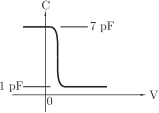The gate oxide thickness in the MOS capacitor is
Explaination / Solution: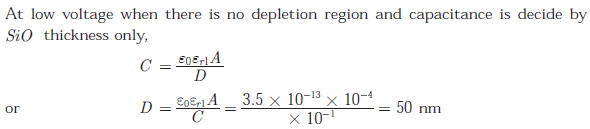Q8. The figure shows the high-frequency capacitance - voltage characteristics of Metal/Sio2/silicon (MOS) capacitor having an area of 1 × 10-4 cm2. Assume that the permittivities (ε0εr) of silicon and Sio are 1 × 10-12 F/cm and 3.5 × 10-13 F/ cm respectively.The maximum depletion layer width in silicon is
Explaination / Solution: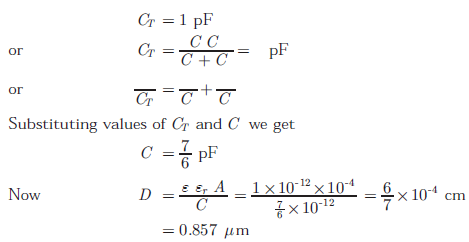Q9.
The figure shows the high-frequency capacitance - voltage characteristics of Metal/Sio2/silicon (MOS) capacitor having an area of 1 × 10-4 cm2. Assume that the permittivities (ε0εr) of silicon and Sio are 1 × 10-12 F/cm and 3.5 × 10-13 F/ cm respectively.Consider the following statements about the C − V characteristics plot :
S1 : The MOS capacitor has as n-type substrate
S2 : If positive charges are introduced in the oxide, the C − V polt will shift to the left.
Then which of the following is true?

Explaination / Solution:

Depletion region will not be formed if the MOS capacitor has n type substrate but from C-V characteristics, C reduces if V is increased. Thus depletion region must be formed. Hence S1 is false If positive charges is introduced in the oxide layer, then to equalize the effect the applied voltage V must be reduced. Thus the C − V plot moves to the left. Hence S2 is true.

Q10. Which of the following is NOT associated with a p - n junction ?
Explaination / Solution:

Channel length modulation is not associated with a p - n junction. It is being associated with MOSFET in which effective channel length decreases, producing the phenomenon called channel length modulation.